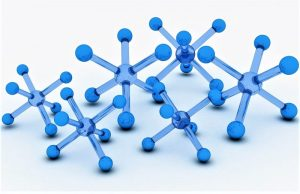Chemistry

# Atomic mass

The substances that we can observe occupy a space and also have mass. Molecules, which are the parts that make up substances, often have to be measured through experiments, and it is important that these measurements are accurate. But how can we measure something so small precisely? How do we normally measure molecules?• Symbol: u o Da
• Unit: Atomic mass unit or Dalton

## What is the atomic mass?

Atomic mass is the mass that an atom has when it is at rest; it is the mass that arises from the total mass of protons and neutrons of a single atom also at rest.

In different laboratories, a tool called analytical balance is used to measure in grams. Through careful calculation, scientists can determine how many moles a specific reaction will need. A mole is a unit of measurement that helps us make comparisons between particles of any given substance and its mass. If we already know how many moles we need, we can use the concept of molar mass to calculate how many grams of the substance are required. Molar mass, also known as molecular weight, is the sum of the total mass in grams of all the atoms that make up a particular molecule. The unit used to measure is grams per mol.

## History

The first scientist to study atomic weight and calculate it was the English chemist John Dalton. He has been known to have developed a theory in this sense that was based on five fundamental points. In addition to John Dalton, Jöns Jakob Berzelius, a Swedish chemist who also made several calculations and who, together with the previous scientist, is considered to be the father of modern chemistry. Polymer, catalysis or isomer were some of the concepts he coined and introduced in this science.

## What the atomic mass represents

Atomic mass represents the number of different isotopes that can be found in the same element, but which differ from each other and is at the same time one of the fundamental properties that atoms have, such as number and mass. Sometimes we find that there is a more common isotope than others, and the difference between their atomic mass and the relative atomic mass or standard atomic weight is small enough not to affect the calculations, but this difference may be important in other cases.

## How it is represented

The atomic mass of the elements is represented by the letter A in the periodic table of the elements. It is represented by the mass number and this number is the one that indicates the total number of particles that can be found in the nucleus, in other words the sum of neutrons and protons. It is in charge of representing the mass that the atom has measured in u.m.a. In some occasions it is also known with the name of atomic weight, however, it is important to mention that this name is incorrect since the mass is a specific property of the body, on the other hand the weight is a characteristic that will depend in its totality on the weight.

## How atomic mass is measured

The unit in which the atomic mass is measured is called just so, atomic mass unit and is represented by the acronym u.m.a. This unit is also called Dalton, and is represented by the word Da, which was given in honor of the chemist John Dalton. The u.m.a corresponds to the twelfth part of the mass of the carbon-12 nucleus, which is the most abundant isotope of this element. The value of a u.m.a is also very close to the mass of a proton. The measurement of the masses of the elements can be carried out by means of a mass spectrometer. The scientific unit for handling atoms in macroscopic quantities is known as a mole.

## How the atomic mass is calculated

Atomic mass can be calculated by adding the numbers of neutrons and the protons or electrons of a given element. They can also be calculated with the weighted measures of the masses of the different isotopes that constitute each element.

## Examples

• Atomic mass of oxygen: In the case of hydrogen, it indicates atomic mass of H = 1.00797, we must read that the mass of an atom of H is 1.00797 times greater than the u.m.a., but not that the mass of an atom of H is 1.00797 g.
• Atomic mass of carbon: Natural carbon is a mixture of three isotopes, 98.892 % of 12 C and 1.108 % of 13 C and a minimum amount of 14 C. Therefore, the average atomic mass of carbon will be: (0.98892) x (12 u.m.a) + (0.01108) x (13.00335 u.m.a) = 12.011 u.m.a.
• Some other atomic masses are: Calcium 087, Cobalt 58,933195, Copper 63,564, Fluorine 18,998403 and iron 55,845.
Written by Gabriela Briceño V.